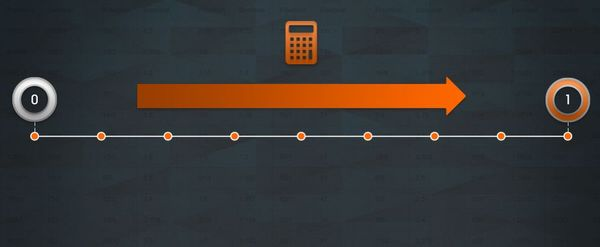# What do betting odds represent

Odds are only a representation of something more important – probability. Getting a handle on how to calculate probability and covert it into decimal odds is the first step in developing your own assessments of betting value.There is a conversation that all seasoned bettors will have had numerous times with friends and relatives who want a casual bet on a major sporting event. What are the odds on x? What do they mean? What will I win if I bet x amount?

Understanding odds is the biggest challenge that anyone new to betting faces. But what do odds actually represent, and how can you understand the return value for a given stake?Once you get past that hurdle, you can compare odds between bookmakers. However, if you consider betting to be just about odds, you’re missing the bigger picture. To really expand your understanding of odds and betting, you must also understand how to calculate probability.

Betting is about assessing the chance or probability of an event happening and bookmakers like Pinnacle use odds to translate probability into a more usable form in order to offer betting.

The fact that there are many different odds formats – decimal, fractional, money line – illustrates the point that odds are simply a means to an end i.e. offering betting. Bookmakers really deal in risk measured by probability.

Considering how we are faced with risk every single day of our lives – what are the chances of me making that train on time? how likely is it to rain? – it is suprising how unfamilair the average person is with being able to calculate probability.

It’s a scale running from 0 – where there is no chance of an event occurring –  to 1 – a certain future event – with the likelihood of all other potential events falling somewhere between those points on the probability spectrum.

A coin-toss is a great way to explain how to calculate probability. The coin will definitely land on either Heads or Tails, which taken together provide us with the certain event, which we now know has a probability of 1.

Of course as a bettor what you really want to know is the probability (or chance) of your chosen call, which we will say is Heads. To do this there is a simple equation.

Favourable outcomes / all possible outcomes

As a bettor you want to know the probability of your chosen call – the favourable outcome – which for this example we will say is Heads. Therefore to get your value divide by the two possible outcomes – Heads or Tails – to produce a probability of 0.5.

In general people are more comfortable with percentages, so by multiplying the probability of your event (0.5 for Heads) by 100 you can say that there is a 50% chance of the coin landing on Heads, and you winning your bet.

Now you know how to calculate probability, you can turn this into odds. Decimal odds are the default format used by bookmakers like Pinnacle, and you can arrive at the decimal odds value for your coin toss choice with the simple equation:

1 / probability for your chosen outcome

So the decimal odds for a coin being Heads is 1 (certainty) divided by the probability of it occurring which we know is 0.5, producing decimal odds of 2.0. At this point you can equally take odds and reverse engineer the implied probability with the inverse of the equation for turning probability into odds:

1/decimal odds = probability

Take your newly found knowledge and work out the implied probability for your coin toss with your friend and you”ll see the aggregate implied probability of both ourcomes in the coin toss is 100% – (0.5/1+0.5/1)*100 – no surprises as a certain event is 1 (100/100).

However, performing the same calculation for actual odds from your favourite bookmaker will produce a value greater than 100%. So what is happening here?

In simple terms the odds don’t reflect the true likelihood – the probability – of the outcomes concerned. The amout by which the implied probability diverges from 100% is the edge your bookmaker holds for that market, and essentially measures the value they are providing.

This is an essential piece of information for a value seeking bettor, is easy to calculate but few if any bookmakers publicly share it – other than Pinnacle Sports. It’s worth asking yourself why that is?

Calclulating odds and probability opens up a new world for calculating value but you also want to know what your bet will payout. For our coin toss example this requires a simple multiplication:

So if you bet \$10 on Heads with odds of 2.0 your return including stake is 2.0 x \$10 which equals \$20 (this includes your \$10 stake + \$10 profit).

Being able to calculate probability and understand where odds actually come from is an essential part of evolving as a bettor because it enables you to calculate your own expected frequency for an event – starting to model your own odds – and then compare what you think will happen with what odds are available.

Where the two diverge you can potentially turn that edge in your favour, and generate profit, which is what a bettor should be focused on.##### MORE: Best E-Sports Betting Sites >>>

Source: pinnacle.com